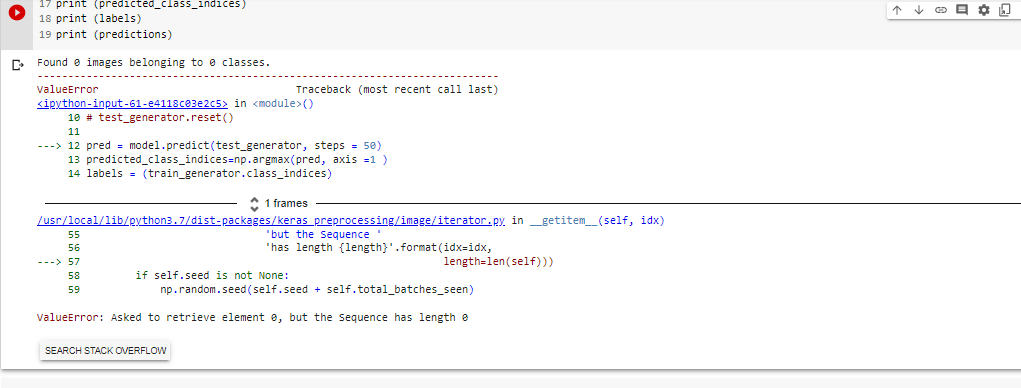Instantly share code, notes, and snippets.

# fchollet/classifier_from_little_data_script_2.py

Last active November 24, 2022 10:01
Updated to the Keras 2.0 API.
This file contains bidirectional Unicode text that may be interpreted or compiled differently than what appears below. To review, open the file in an editor that reveals hidden Unicode characters. Learn more about bidirectional Unicode characters
 '''This script goes along the blog post "Building powerful image classification models using very little data" from blog.keras.io. It uses data that can be downloaded at: https://www.kaggle.com/c/dogs-vs-cats/data In our setup, we: - created a data/ folder - created train/ and validation/ subfolders inside data/ - created cats/ and dogs/ subfolders inside train/ and validation/ - put the cat pictures index 0-999 in data/train/cats - put the cat pictures index 1000-1400 in data/validation/cats - put the dogs pictures index 12500-13499 in data/train/dogs - put the dog pictures index 13500-13900 in data/validation/dogs So that we have 1000 training examples for each class, and 400 validation examples for each class. In summary, this is our directory structure: ``` data/ train/ dogs/ dog001.jpg dog002.jpg ... cats/ cat001.jpg cat002.jpg ... validation/ dogs/ dog001.jpg dog002.jpg ... cats/ cat001.jpg cat002.jpg ... ``` ''' import numpy as np from keras.preprocessing.image import ImageDataGenerator from keras.models import Sequential from keras.layers import Dropout, Flatten, Dense from keras import applications # dimensions of our images. img_width, img_height = 150, 150 top_model_weights_path = 'bottleneck_fc_model.h5' train_data_dir = 'data/train' validation_data_dir = 'data/validation' nb_train_samples = 2000 nb_validation_samples = 800 epochs = 50 batch_size = 16 def save_bottlebeck_features(): datagen = ImageDataGenerator(rescale=1. / 255) # build the VGG16 network model = applications.VGG16(include_top=False, weights='imagenet') generator = datagen.flow_from_directory( train_data_dir, target_size=(img_width, img_height), batch_size=batch_size, class_mode=None, shuffle=False) bottleneck_features_train = model.predict_generator( generator, nb_train_samples // batch_size) np.save(open('bottleneck_features_train.npy', 'w'), bottleneck_features_train) generator = datagen.flow_from_directory( validation_data_dir, target_size=(img_width, img_height), batch_size=batch_size, class_mode=None, shuffle=False) bottleneck_features_validation = model.predict_generator( generator, nb_validation_samples // batch_size) np.save(open('bottleneck_features_validation.npy', 'w'), bottleneck_features_validation) def train_top_model(): train_data = np.load(open('bottleneck_features_train.npy')) train_labels = np.array(  * (nb_train_samples / 2) +  * (nb_train_samples / 2)) validation_data = np.load(open('bottleneck_features_validation.npy')) validation_labels = np.array(  * (nb_validation_samples / 2) +  * (nb_validation_samples / 2)) model = Sequential() model.add(Flatten(input_shape=train_data.shape[1:])) model.add(Dense(256, activation='relu')) model.add(Dropout(0.5)) model.add(Dense(1, activation='sigmoid')) model.compile(optimizer='rmsprop', loss='binary_crossentropy', metrics=['accuracy']) model.fit(train_data, train_labels, epochs=epochs, batch_size=batch_size, validation_data=(validation_data, validation_labels)) model.save_weights(top_model_weights_path) save_bottlebeck_features() train_top_model()

### Fine tuned models' Prediction code

This codes were checked by myself. They all worked fine.

1. If someone want to predict image classes in same model script where model were trained, here is the code :
``````img_width, img_height = 224, 224
batch_size = 1

datagen = ImageDataGenerator(rescale=1. / 255)

test_generator = datagen.flow_from_directory(
test_dir,
target_size=(img_width, img_height),
batch_size=batch_size,
class_mode=None,
shuffle=False)

test_generator.reset()

pred= model.predict_generator(test_generator, steps = no_of_images/batch_size)
predicted_class_indices=np.argmax(pred, axis =1 )
labels = (train_generator.class_indices)
labels = dict((v, k) for k, v in labels.items())
predictions = [labels[k] for k in predicted_class_indices]
print(predicted_class_indices)
print (labels)
print (predictions)
``````

1. If someone want to predict image classes in different script (separate from training script file), here is the code :
``````from tensorflow.python.keras.preprocessing.image import ImageDataGenerator
import json
import os
from tensorflow.keras.models import model_from_json

#Just give below lines parameters
best_weights = 'path to .h5 weight file'
model_json = 'path to saved model json file'
test_dir =  'path to test images'

img_width, img_height = 224, 224
batch_size = 1
nb_img_samples = #no of testing images

with open(model_json, 'r') as json_file:

model = tf.keras.models.model_from_json(json_savedModel)

model.summary()

datagen = ImageDataGenerator(rescale=1. / 255)

test_generator = datagen.flow_from_directory(
folder_path,
target_size=(img_width, img_height),
batch_size=batch_size,
class_mode=None,
shuffle=False)

test_generator.reset()

pred= model.predict_generator(test_generator, steps = nb_img_samples/batch_size)
predicted_class_indices=np.argmax(pred,axis=1)
labels = {'cats': 0, 'dogs': 1} #if you have more classes, just add like this in correct order where your training folder order.
labels = dict((v,k) for k,v in labels.items())
predictions = [labels[k] for k in predicted_class_indices]
print(predicted_class_indices)
print (labels)
print (predictions)
``````please help me, I got this error when running that codes, I want to predict image classes in same model script